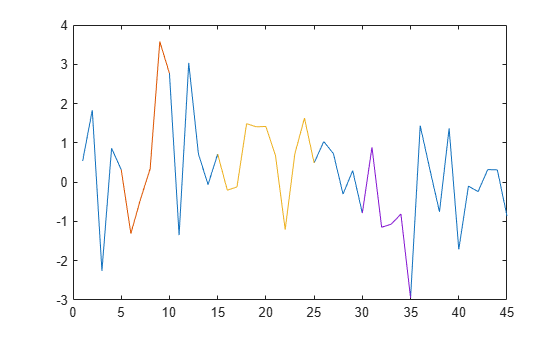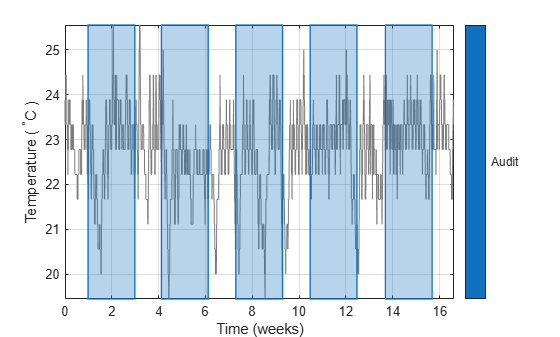# extractsigroi

Extract signal regions of interest

## Syntax

``sigroi = extractsigroi(x,roilims)``
``sigroi = extractsigroi(x,roilims,concat)``

## Description

example

````sigroi = extractsigroi(x,roilims)` extracts regions of interest (ROIs) of the input signal vector `x` based on the ROI limits specified in `roilims`.```

example

````sigroi = extractsigroi(x,roilims,concat)` with `concat` specified as `true` extracts regions of interest and concatenates them.```

## Examples

collapse all

Consider a two-column matrix representing possible regions of interest of a 45-sample random signal. Extract the signal samples corresponding to the regions of interest.

```x = randn(45,1); roilims = [5 10; 15 25; 30 35]; sigroi = extractsigroi(x,roilims);```

Plot the signal and highlight the regions of interest.

```plot(x) hold on for kj = 1:length(sigroi) plot(roilims(kj,1):roilims(kj,2),sigroi{kj}) end hold off```Consider a set of temperature data collected by a thermometer inside an office building for about four months. The device takes a reading every half hour. The sample rate is thus 48 measurements/day. Convert the temperature to degrees Celsius and plot the data.

```load officetemp tempC = (temp-32)*5/9; fs = 48; t = (0:length(tempC) - 1)/fs; plot(t,tempC) xlabel('Time (days)') ylabel('Temperature ( {}^\circC )')```Create region-of-interest limits that separate the temperature data into 29-day periods.

`roilims = [1 29; 30 58; 59 87; 88 116];`

Extract the regions of interest. Compute the mean temperature of each period and display the values.

```sigroi = extractsigroi(tempC,roilims*fs); cellfun(@mean,sigroi)'```
```ans = 1×4 22.8819 22.3073 22.7633 23.0066 ```

Consider a two-column matrix representing possible regions of interest of a 45-sample random signal. Extract the signal samples corresponding to the regions of interest. Concatenate the samples into a single vector.

```x = randn(45,1); roilims = [5 10; 15 25; 30 35]; sigroi = extractsigroi(x,roilims,true);```

Plot the signal and highlight the regions of interest.

```plot(x) y = NaN(size(x)); for kj = 1:size(roilims,1) roi = roilims(kj,1):roilims(kj,2); y(roi) = sigroi(1:length(roi)); sigroi(1:length(roi)) = []; end hold on plot(y) hold off```Consider a set of temperature data collected by a thermometer inside an office building for four months. The device takes a reading every half hour. The sample rate is thus 48 measurements/day. Convert the temperature to degrees Celsius.

```load officetemp tempC = (temp-32)*5/9; fs = 48;```

Create region-of-interest (ROI) limits that correspond to five random two-week periods separated by at least 24 hours. Use the temperature readings from these days for an audit.

```r = 5; w = 14*fs; s = 1*fs; hq = histcounts(randi(r+1,1,length(tempC)-r*w-(r-1)*s),(1:r+2)-1/2); t = (1 + (0:r-1)*(w+s) + cumsum(hq(1:r)))'; roilims = [t t+w-1];```

Extract the regions of interest. Compute the mean temperature of each audited region of interest and display the values.

```sigroi = extractsigroi(tempC,roilims); cellfun(@mean,sigroi)'```
```ans = 1×5 22.8075 22.2586 22.4256 22.9018 23.1457 ```

Extract the regions of interest again, but now concatenate the samples into a single vector. Compute the mean temperature across the audited regions.

```sigroic = extractsigroi(tempC,roilims,true); avgTFc = mean(sigroic)```
```avgTFc = 22.7078 ```

Convert the ROI limits to a binary sequence and create a mask. Express time in weeks.

```m = sigroi2binmask(roilims,length(tempC)); msk = signalMask(m,'SampleRate',fs*7,'Categories',"Audit");```

Plot the data and visualize the regions of interest with rectangular patches.

```plotsigroi(msk,tempC,true) xlabel('Time (weeks)') ylabel('Temperature ( {}^\circC )')```## Input Arguments

collapse all

Input signal, specified as a vector.

Example: `chirp(0:1/1e3:1,25,1,50)` specifies a chirp sampled at 1 kHz.

Data Types: `single` | `double`
Complex Number Support: Yes

Region-of-interest limits, specified as a two-column matrix of positive integers. The ith row of `roilims` contains nondecreasing indices corresponding to the beginning and end samples of the ith region of interest of a signal.

Example: `[5 8; 12 20; 18 25]` specifies a two-column region-of-interest matrix with three regions.

Data Types: `single` | `double` | `int8` | `int16` | `int32` | `int64` | `uint8` | `uint16` | `uint32` | `uint64`

Option to concatenate extracted signal regions, specified as a logical value.

Data Types: `logical`

## Output Arguments

collapse all

Signal regions of interest, returned as a cell array or a vector.

• If `concat` is set to `false`, `sigroi` is a cell array. The ith cell of `sigroi` contains the signal samples corresponding to the ith region of interest specified in `roilims`.

• If `concat` is set to `true`, `sigroi` is a vector that concatenates all extracted signal samples.

## Extended Capabilities

Introduced in R2020b